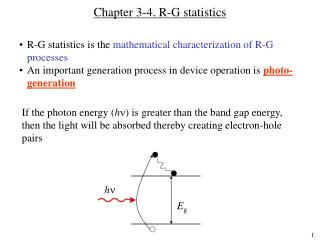# Chapter 3-4. R-G statistics - PowerPoint PPT PresentationDownload PresentationChapter 3-4. R-G statistics

Chapter 3-4. R-G statisticsDownload Presentation## Chapter 3-4. R-G statistics

- - - - - - - - - - - - - - - - - - - - - - - - - - - E N D - - - - - - - - - - - - - - - - - - - - - - - - - - -
##### Presentation Transcript

1. Chapter 3-4. R-G statistics • R-G statistics is the mathematical characterization of R-G processes • An important generation process in device operation is photo-generation If the photon energy (h) is greater than the band gap energy, then the light will be absorbed thereby creating electron-hole pairs h Eg

2. Photo-generation The intensity of monochromatic light that passes through a material is given by: I = I0 exp(  x) where I0is the light intensity just inside the material at x = 0, and  is the absorption coefficient. Note that  is material dependent and is a strong function of . Since photo-generation creates equal #s of holes and electrons, and each photon creates one e-h pair, we can write: where GL0 is the photo-generation rate [# / (cm3 s)] at x = 0 Question: What happens if the energy of photons is less than the band gap energy?

3. Light absorption and transmittance Consider a slab of semiconductor of thickness l. l h h I0 It semiconductor x 0 l It = I0 exp (l ) where I0 is light intensity at x = 0 and It is light intensity at x = l.

4. Absorption coefficient vs. wavelength in semiconductors

5. Bandgaps of common semiconductors

6. Indirect thermal recombination-generation • n0, p0 - under thermal equilibrium • n, p - under arbitrary conditions, functions of t • n = n – n0 • p = p – p0 • Nt is the # of R-G centers/cm3 • Low-level injection condition is assumed. • Change in the majority carrier concentration is negligible. For example, in n-type material, p << n0 ; n  n0. n and pare deviations in carrier concentrations from their equilibrium values. n and p can be both positive or negative.

7. R-G statistics Consider n-type silicon under perturbation: We look at only minority carriers, and in this case, holes. In general, rate of hole build up (loss) due to recomb. (gain) due to generation external such as light should be proportional to p and Nt. Why?

8. R-G statistics (continued) Under thermal equilibrium, GL = 0; and dp/dt = 0 So, under arbitrary conditions, when GL = 0,

9. R-G statistics (continued) We can write: For holes in n-type Similarly, For electrons in p-type p (or n) is called “minority carrier lifetime”indicating the average time an excess minority carrier will survive in a sea of majority carriers. Minority carrier lifetime is an important material parameter. Depends strongly on the concentration of deep-level of impurities, crystalline quality etc.. Varies strongly from material to material. Varies from a few ns to few ms in silicon depending on the quality!

10. What happens when the perturbation is removed at t = 0? For holes in an n-type semiconductor p The solution for t > 0 is: p(0) t The excess carrier concentration exponentially decays to zero when the external perturbation is removed. This fact is used to measure lifetimes using photo-conductivity decay technique. See Sect. 3.3.4.

11. Photoconductivity decay measurement

12. Photoconductivity decay measurement system

13. Photoconductivity transient response